Water reservoir

One drain hole emptied the water from the tank for 20 hours, with the second drain for 30 hours. How long will the water drain from this tank if we open two drains simultaneously?

Result

t =  12 h

Solution:

t*(1/20 + 1/30)=1

5t = 60

t = 12

Calculated by our simple equation calculator.

Leave us a comment of this math problem and its solution (i.e. if it is still somewhat unclear...):Be the first to comment!To solve this verbal math problem are needed these knowledge from mathematics:

Looking for help with calculating harmonic mean? Looking for a statistical calculator? Need help calculate sum, simplify or multiply fractions? Try our fraction calculator. Do you have a linear equation or system of equations and looking for its solution? Or do you have quadratic equation?

Next similar math problems:

1. Hectoliters of waterThe pool has a total of 126 hectoliters of water. The first pump draws 2.1 liters of water per second. A second pump pumps 3.5 liters of water per second. How long will it take both pumps to drain four-fifths of the water at the same time?
2. Theorem proveWe want to prove the sentence: If the natural number n is divisible by six, then n is divisible by three. From what assumption we started?
3. Two pumps togetherThe first pump will fill the tank itself in 3 hours and the second one in 6 hours. How many hours will the tank be full if both pumps are worked at the same time?
4. Simple equation 6Solve equation with one variable: X/2+X/3+X/4=X+4
5. Working togetherOne painter paint the nursery in 10 days, the second in 8 days. How many days will the nursery be painted if they work together?
6. Painters 4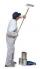One painter will do the job for 12 days. After a 4 1/3 day he comes second to help him and complete work together for 8 days. How long would work the second painter himself?
7. Two typists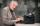There are two typists who are rewriting the material 814 pages. First can it handle rewrite yourself for 24 days; the second 12 days. First typist wrote material yourself 4 days rest rewrites yourself second typist. How many days will it take rewriting alt
8. Painters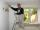The first team of painters would paint bridge in 15 days, the second in 10 days. After 3 days of working together second team goes out and continue only first team. How many days took second team to finish painting the bridge?
9. Six workersSix workers planned to repair the roof in 5 days. After two days, two workers get sick. How long will the remaining workers complete the roof at the same rate of work?
10. Minute averageIn a factory, four workers are assigned to complete an order received for dispatching 1400 boxes of a particular commodity. Worker A takes 4 mins per box, Worker B takes 6 minutes per box, C takes 10 mins per box, D takes 15 mins per box. Find the average.
11. Invitations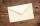For every 5 minutes writes Dana 10 invitations, while Anna 14 invitations. How long will write together 120 invitations?
12. Tonic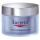One package tonic consume Mom 12 weeks and daughter for 14 weeks. 4 weeks they use the same cream together and then only daughter. How many weeks endured tonic to daughter then?
13. Digging companies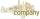Company A would dig a pit for 12 days, company B for 15 days, company C for 20 days and the company D for 24 days. Work began together companies C and D, but after three days joined them other two companies. How long took to dig a pit?
14. Fifth of the numberThe fifth of the number is by 24 less than that number. What is the number?
15. Six yearsIn six years Jan will be twice as old as he was six years ago. How old is he?
16. Bricklayers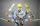When a bricklayer works himself to redeem the house in 8 days, the other bricklayer will be finished in 10 days. How long will it take to make 3 such houses together?
17. Our houseOur house would paint four painters in 14 days. How long could it paint five painters? How long will it take if two other painters will come to help after 5 days?